Courses

# Introduction to Letter Series Notes | EduRev

## UPSC : Introduction to Letter Series Notes | EduRev

The document Introduction to Letter Series Notes | EduRev is a part of the UPSC Course UPSC CSAT Preparation.
All you need of UPSC at this link: UPSC

Letter Series

The concept of letter series is related to 26 letters of the alphabet series.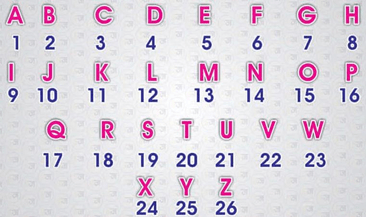The questions asked would vary based on the position of an English letter to the left or right of another English letter in the alphabetical order. Sometimes the questions are asked on finding the number of English letter(s) between two different English letters, and sometimes on the arrangement of alphabetical order. It can be forward, first half forward, second half forward, backward, first half backward, second half backward, multiple letter segments in changed order, word formation, and letter position in word or series etc.

Important points to keep in mind always while solving letter series questions
(i) Position number of letters in English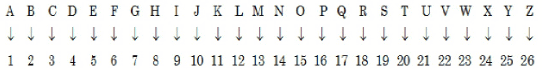(ii) Position number of letters in English alphabets in reverse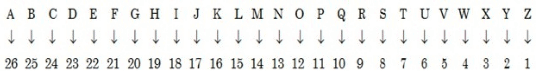(iii) A, E , I, O and U are Vowels of English Alphabets and the remaining letters are Consonants of English Alphabets.
(iv) A → M (A to M) letters are called first half of English Alphabets.
(v) N → Z (N to Z) letters are called second half of English Alphabets.
(vi) From A → Z (A to Z) letters are called 'left to right' or 'to right' in English Alphabets.
(vii) From Z → A (Z to A) letters are called 'right to left' or 'to right' in English Alphabets.
It is important to note that while solving the questions of letter series, if you keep in mind the forward and backward position of all the alphabets along with its number, you can save a lot of time.

The following two concepts in order to recall the position of alphabets easily.
1. Concept of EJOTY

Concept of EJOTY represents the position of the alphabets counting from the left at an interval of 5 letters: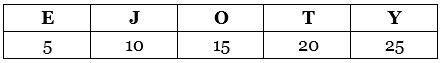Each letter in the term EJOTY represents the position which is multiple of 5.

2. Concept of VQLGB

Just like the concept EJOTY, the concept of VQLGB represents the position of the alphabets counting from the right at an interval of 5 letters: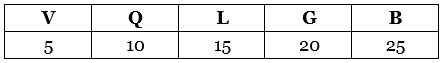In the next tutorials, we will talk about the different categories of questions that can be asked on letter series.

Practice Questions
Q.1. Find the letter on following positions:
(a) 17
(b) 23
(c) 7
(d) 12

Ans.
(a) 17 = 15(O) + 2 = O + 2 = Q
(b) 23 = 20(T) + 3 = T + 3 =W
(c) 7   = 5(E) + 2 = E + 2 = G
(d) 12 = 10(J) + 2 = J + 2 = L

The above all positions of the letters are from left hand side of the series. One must also know the positions of letters from the right hand side of the series also. Let us check how we will do this:

For example:
We know that Letter “A” is on 1st position from left side and “26” form the right hand side. Therefore, the sum of the positions of any letter from both sides will always be 27.
Therefore, to check any position from right hand side, we will first convert that position to left hand side by deducting it from 27.

Q.2. Find the letters on the following positions from right hand side of   the alphabet series:
(a) 17
(b) 23
(c) 7
(d) 12

To find out these positions we will first convert all the positions from the left hand side of the series:
Ans.
(a) 17th from the right means (27-17) = 10th from left = J
(b) 23rd from the right means (27-23) = 4th from left = D
(c) 7th from the right means (27-7) = 20th from left = T
(d) 12th from the right means (27-12) = 15th from left = O

Offer running on EduRev: Apply code STAYHOME200 to get INR 200 off on our premium plan EduRev Infinity!

## UPSC CSAT Preparation

44 videos|64 docs|90 tests

,

,

,

,

,

,

,

,

,

,

,

,

,

,

,

,

,

,

,

,

,

;